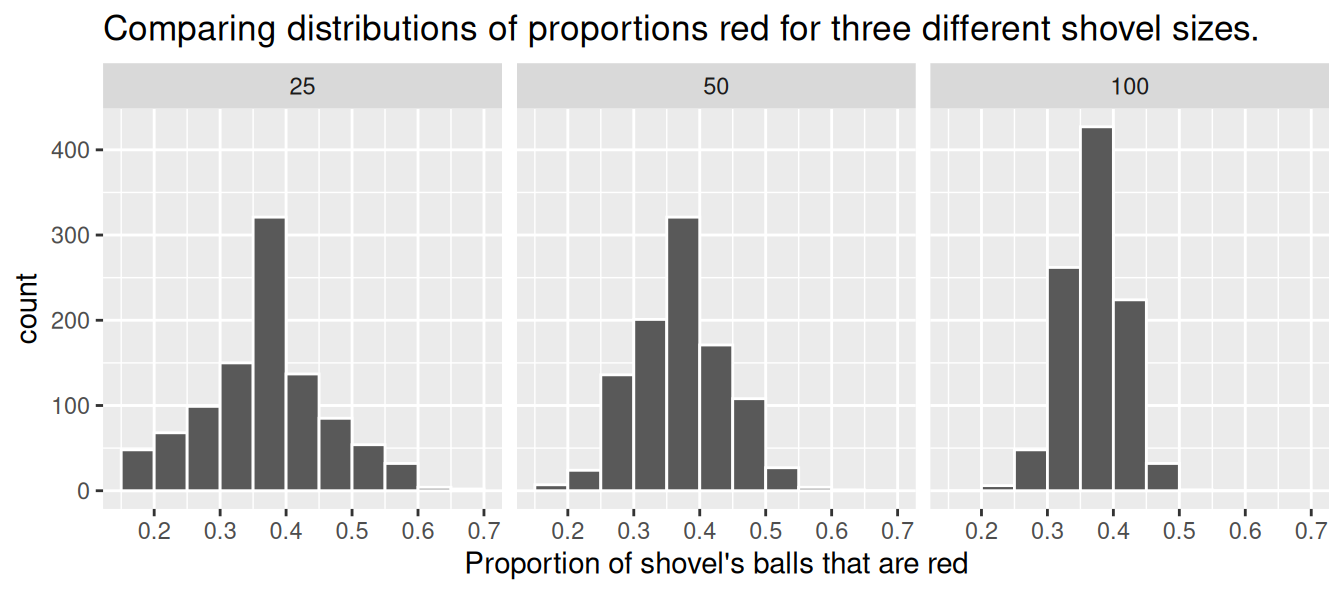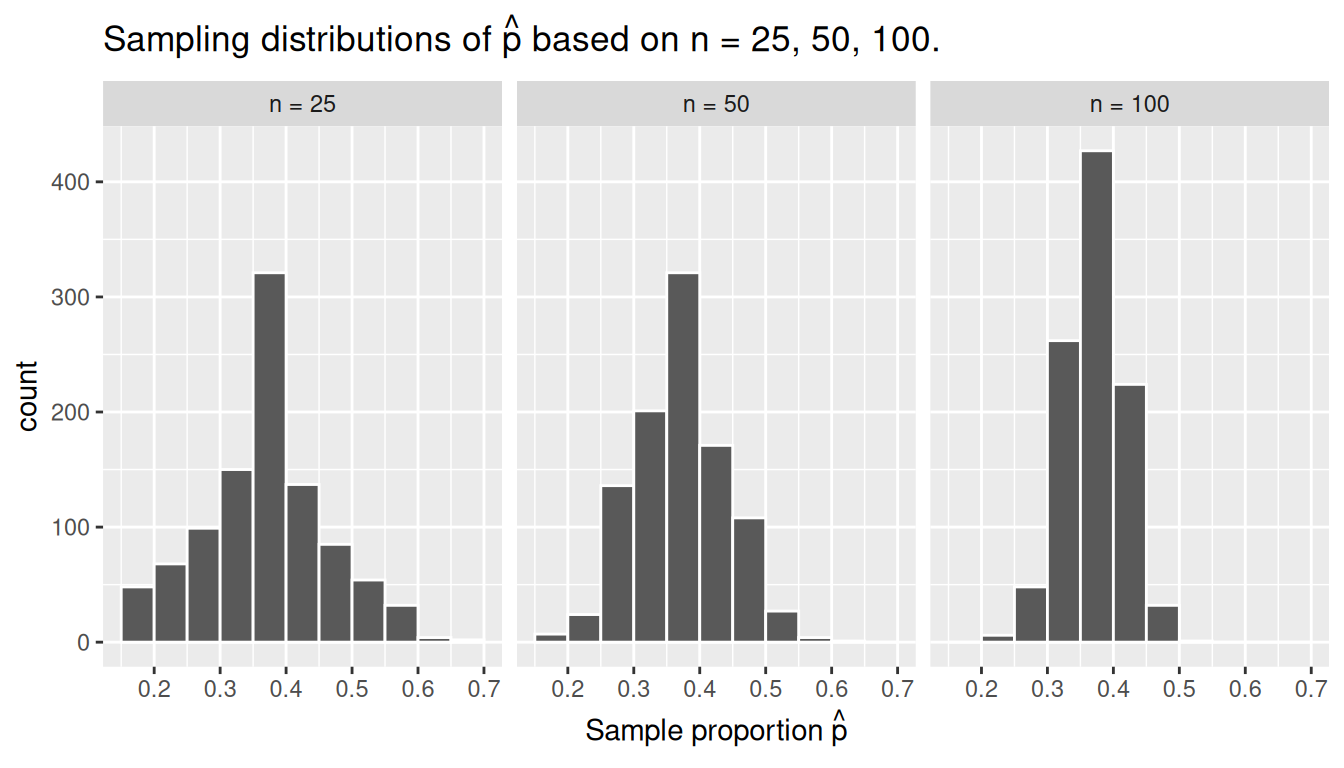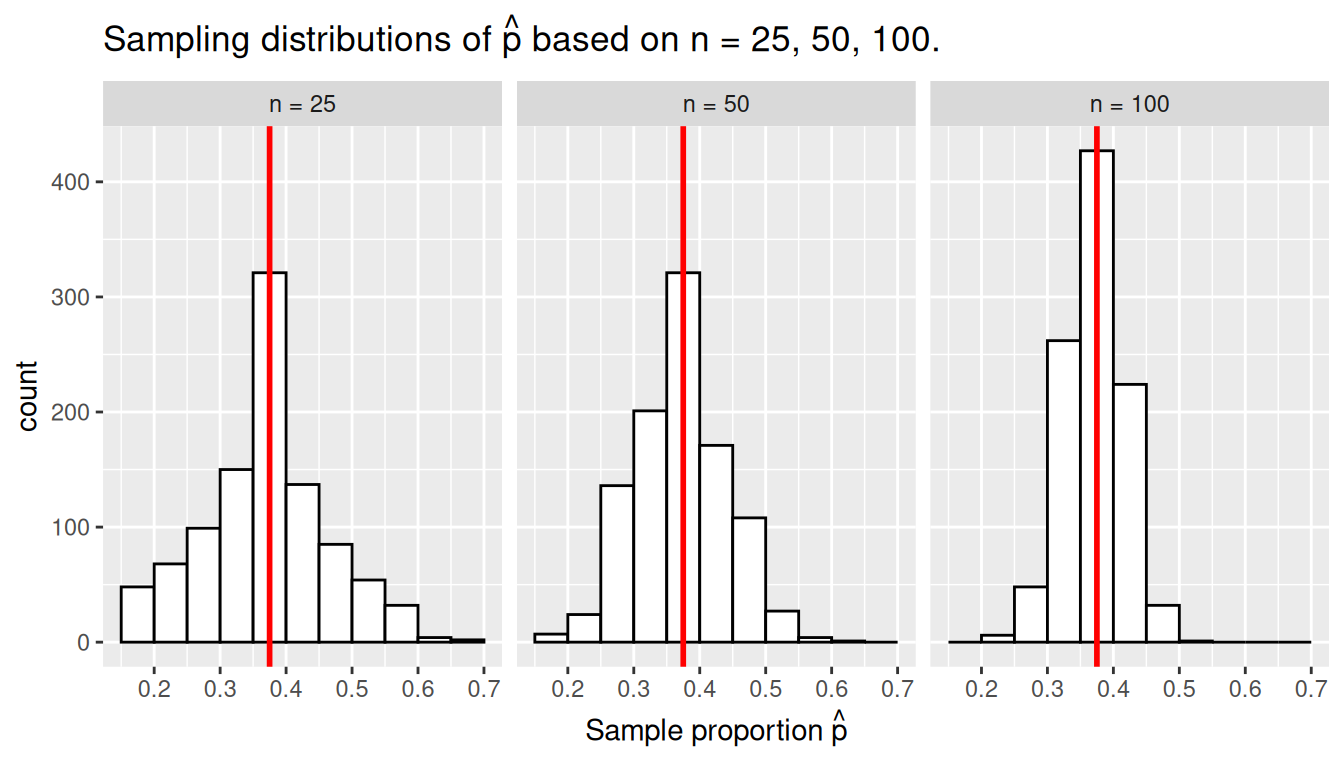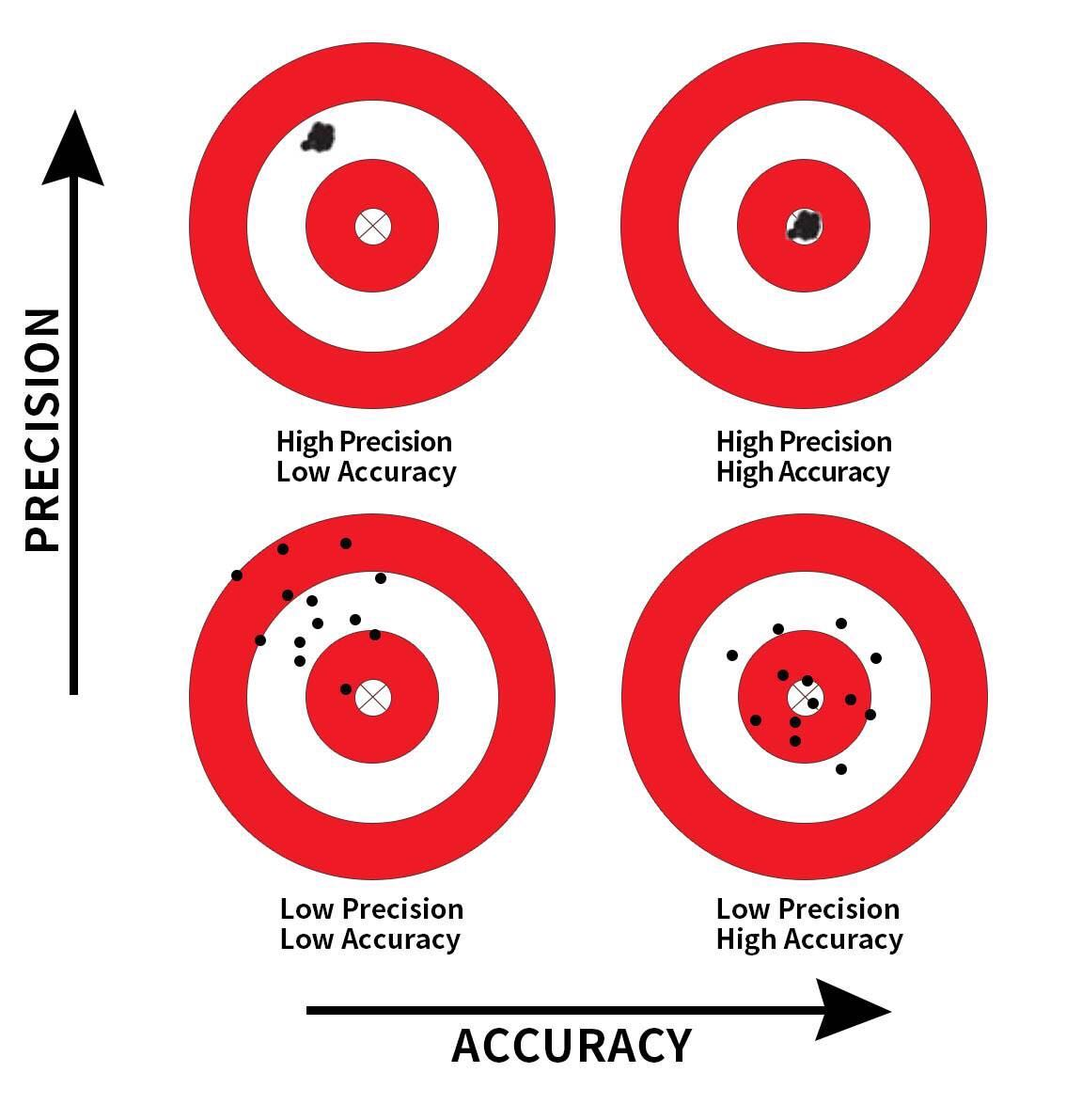## 7.3 Sampling framework

In both our tactile and our virtual sampling activities, we used sampling for the purpose of estimation. We extracted samples in order to estimate the proportion of the bowl’s balls that are red. We used sampling as a less time-consuming approach than performing an exhaustive count of all the balls. Our virtual sampling activity built up to the results shown in Figure 7.12 and Table 7.1: comparing 1000 proportions red based on samples of size 25, 50, and 100. This was our first attempt at understanding two key concepts relating to sampling for estimation:

1. The effect of sampling variation on our estimates.
2. The effect of sample size on sampling variation.

Let’s now introduce some terminology and notation as well as statistical definitions related to sampling. Given the number of new words you’ll need to learn, you will likely have to read this section a few times. Keep in mind, however, that all of the concepts underlying these terminology, notation, and definitions tie directly to the concepts underlying our tactile and virtual sampling activities. It will simply take time and practice to master them.

### 7.3.1 Terminology and notation

Here is a list of terminology and mathematical notation relating to sampling.

First, a population is a collection of individuals or observations we are interested in. This is also commonly denoted as a study population. We mathematically denote the population’s size using upper-case $$N$$. In our sampling activities, the (study) population is the collection of $$N$$ = 2400 identically sized red and white balls contained in the bowl.

Second, a population parameter is a numerical summary quantity about the population that is unknown, but you wish you knew. For example, when this quantity is a mean, the population parameter of interest is the population mean. This is mathematically denoted with the Greek letter $$\mu$$ pronounced “mu” (we’ll see a sampling activity involving means in the upcoming Section 8.1). In our earlier sampling from the bowl activity, however, since we were interested in the proportion of the bowl’s balls that were red, the population parameter is the population proportion. This is mathematically denoted with the letter $$p$$.

Third, a census is an exhaustive enumeration or counting of all $$N$$ individuals or observations in the population in order to compute the population parameter’s value exactly. In our sampling activity, this would correspond to counting the number of balls out of $$N$$ = 2400 that are red and computing the population proportion $$p$$ that are red exactly. When the number $$N$$ of individuals or observations in our population is large as was the case with our bowl, a census can be quite expensive in terms of time, energy, and money.

Fourth, sampling is the act of collecting a sample from the population when we don’t have the means to perform a census. We mathematically denote the sample’s size using lower case $$n$$, as opposed to upper case $$N$$ which denotes the population’s size. Typically the sample size $$n$$ is much smaller than the population size $$N$$. Thus sampling is a much cheaper alternative than performing a census. In our sampling activities, we used shovels with 25, 50, and 100 slots to extract samples of size $$n$$ = 25, $$n$$ = 50, and $$n$$ = 100.

Fifth, a point estimate (AKA sample statistic) is a summary statistic computed from a sample that estimates an unknown population parameter. In our sampling activities, recall that the unknown population parameter was the population proportion and that this is mathematically denoted with $$p$$. Our point estimate is the sample proportion: the proportion of the shovel’s balls that are red. In other words, it is our guess of the proportion of the bowl’s balls that are red. We mathematically denote the sample proportion using $$\widehat{p}$$. The “hat” on top of the $$p$$ indicates that it is an estimate of the unknown population proportion $$p$$.

Sixth is the idea of representative sampling. A sample is said to be a representative sample if it roughly looks like the population. In other words, are the sample’s characteristics a good representation of the population’s characteristics? In our sampling activity, are the samples of $$n$$ balls extracted using our shovels representative of the bowl’s $$N$$ = 2400 balls?

Seventh is the idea of generalizability. We say a sample is generalizable if any results based on the sample can generalize to the population. In other words, does the value of the point estimate generalize to the population? In our sampling activity, can we generalize the sample proportion from our shovels to the entire bowl? Using our mathematical notation, this is akin to asking if $$\widehat{p}$$ is a “good guess” of $$p$$?

Eighth, we say biased sampling occurs if certain individuals or observations in a population have a higher chance of being included in a sample than others. We say a sampling procedure is unbiased if every observation in a population had an equal chance of being sampled. In our sampling activities, since we mixed all $$N = 2400$$ balls prior to each group’s sampling and since each of the equally sized balls had an equal chance of being sampled, our samples were unbiased.

Ninth and lastly, the idea of random sampling. We say a sampling procedure is random if we sample randomly from the population in an unbiased fashion. In our sampling activities, this would correspond to sufficiently mixing the bowl before each use of the shovel.

Phew, that’s a lot of new terminology and notation to learn! Let’s put them all together to describe the paradigm of sampling.

In general:

• If the sampling of a sample of size $$n$$ is done at random, then
• the sample is unbiased and representative of the population of size $$N$$, thus
• any result based on the sample can generalize to the population, thus
• the point estimate is a “good guess” of the unknown population parameter, thus
• instead of performing a census, we can infer about the population using sampling.

Specific to our sampling activity:

• If we extract a sample of $$n=50$$ balls at random, in other words, we mix all of the equally sized balls before using the shovel, then
• the contents of the shovel are an unbiased representation of the contents of the bowl’s 2400 balls, thus
• any result based on the shovel’s balls can generalize to the bowl, thus
• the sample proportion $$\widehat{p}$$ of the $$n=50$$ balls in the shovel that are red is a “good guess” of the population proportion $$p$$ of the $$N=2400$$ balls that are red, thus
• instead of manually going over all 2400 balls in the bowl, we can infer about the bowl using the shovel.

Note that last word we wrote in bold: infer. The act of “inferring” means to deduce or conclude information from evidence and reasoning. In our sampling activities, we wanted to infer about the proportion of the bowl’s balls that are red. Statistical inference is the “theory, methods, and practice of forming judgments about the parameters of a population and the reliability of statistical relationships, typically on the basis of random sampling.” In other words, statistical inference is the act of inference via sampling. In the upcoming Chapter 8 on confidence intervals, we’ll introduce the infer package, which makes statistical inference “tidy” and transparent. It is why this third portion of the book is called “Statistical inference via infer.”

Learning check

(LC7.8) In the case of our bowl activity, what is the population parameter? Do we know its value?

(LC7.9) What would performing a census in our bowl activity correspond to? Why did we not perform a census?

(LC7.10) What purpose do point estimates serve in general? What is the name of the point estimate specific to our bowl activity? What is its mathematical notation?

(LC7.11) How did we ensure that our tactile samples using the shovel were random?

(LC7.12) Why is it important that sampling be done at random?

(LC7.13) What are we inferring about the bowl based on the samples using the shovel?

### 7.3.2 Statistical definitions

Now, for some important statistical definitions related to sampling. As a refresher of our 1000 repeated/replicated virtual samples of size $$n$$ = 25, $$n$$ = 50, and $$n$$ = 100 in Section 7.2, let’s display Figure 7.12 again as Figure 7.13.FIGURE 7.13: Previously seen three distributions of the sample proportion $$\widehat{p}$$.

These types of distributions have a special name: sampling distributions; their visualization displays the effect of sampling variation on the distribution of any point estimate, in this case, the sample proportion $$\widehat{p}$$. Using these sampling distributions, for a given sample size $$n$$, we can make statements about what values we can typically expect.

For example, observe the centers of all three sampling distributions: they are all roughly centered around $$0.4 = 40\%$$. Furthermore, observe that while we are somewhat likely to observe sample proportions of red balls of $$0.2 = 20\%$$ when using the shovel with 25 slots, we will almost never observe a proportion of 20% when using the shovel with 100 slots. Observe also the effect of sample size on the sampling variation. As the sample size $$n$$ increases from 25 to 50 to 100, the variation of the sampling distribution decreases and thus the values cluster more and more tightly around the same center of around 40%. We quantified this variation using the standard deviation of our sample proportions in Table 7.1, which we display again as Table 7.2:

TABLE 7.2: Previously seen comparing standard deviations of proportions red for three different shovels
Number of slots in shovel Standard deviation of proportions red
25 0.094
50 0.069
100 0.045

So as the sample size increases, the standard deviation of the proportion of red balls decreases. This type of standard deviation has another special name: standard error. Standard errors quantify the effect of sampling variation induced on our estimates. In other words, they quantify how much we can expect different proportions of a shovel’s balls that are red to vary from one sample to another sample to another sample, and so on. As a general rule, as sample size increases, the standard error decreases.

Unfortunately, these names confuse many people who are new to statistical inference. For example, it’s common for people who are new to statistical inference to call the “sampling distribution” the “sample distribution.” Another additional source of confusion is the name “standard deviation” and “standard error.” Remember that a standard error is merely a kind of standard deviation: the standard deviation of any point estimate from sampling. In other words, all standard errors are standard deviations, but not every standard deviation is necessarily a standard error.

To help reinforce these concepts, let’s re-display Figure 7.12 but using our new terminology, notation, and definitions relating to sampling in Figure 7.14.FIGURE 7.14: Three sampling distributions of the sample proportion $$\widehat{p}$$.

Furthermore, let’s re-display Table 7.1 but using our new terminology, notation, and definitions relating to sampling in Table 7.3.

TABLE 7.3: Standard errors of the sample proportion based on sample sizes of 25, 50, and 100
Sample size (n) Standard error of $$\widehat{p}$$
n = 25 0.094
n = 50 0.069
n = 100 0.045

Remember the key message of this last table: that as the sample size $$n$$ goes up, the “typical” error of your point estimate will go down, as quantified by the standard error.

Learning check

(LC7.14) What purpose did the sampling distributions serve?

(LC7.15) What does the standard error of the sample proportion $$\widehat{p}$$ quantify?

### 7.3.3 The moral of the story

Let’s recap this section so far. We’ve seen that if a sample is generated at random, then the resulting point estimate is a “good guess” of the true unknown population parameter. In our sampling activities, since we made sure to mix the balls first before extracting a sample with the shovel, the resulting sample proportion $$\widehat{p}$$ of the shovel’s balls that were red was a “good guess” of the population proportion $$p$$ of the bowl’s balls that were red.

However, what do we mean by our point estimate being a “good guess”? Sometimes, we’ll get an estimate that is less than the true value of the population parameter, while at other times we’ll get an estimate that is greater. This is due to sampling variation. However, despite this sampling variation, our estimates will “on average” be correct and thus will be centered at the true value. This is because our sampling was done at random and thus in an unbiased fashion.

In our sampling activities, sometimes our sample proportion $$\widehat{p}$$ was less than the true population proportion $$p$$, while at other times it was greater. This was due to the sampling variability. However, despite this sampling variation, our sample proportions $$\widehat{p}$$ were “on average” correct and thus were centered at the true value of the population proportion $$p$$. This is because we mixed our bowl before taking samples and thus the sampling was done at random and thus in an unbiased fashion. This is also known as having an accurate estimate.

What was the value of the population proportion $$p$$ of the $$N$$ = 2400 balls in the actual bowl that were red? There were 900 red balls, for a proportion red of 900/2400 = 0.375 = 37.5%! How do we know this? Did the authors do an exhaustive count of all the balls? No! They were listed in the contents of the box that the bowl came in! Hence we were able to make the contents of the virtual bowl match the tactile bowl:

bowl %>%
summarize(sum_red = sum(color == "red"),
sum_not_red = sum(color != "red"))
# A tibble: 1 x 2
sum_red sum_not_red
<int>       <int>
1     900        1500

Let’s re-display our sampling distributions from Figures 7.12 and 7.14, but now with a vertical red line marking the true population proportion $$p$$ of balls that are red = 37.5% in Figure 7.15. We see that while there is a certain amount of error in the sample proportions $$\widehat{p}$$ for all three sampling distributions, on average the $$\widehat{p}$$ are centered at the true population proportion red $$p$$.FIGURE 7.15: Three sampling distributions with population proportion $$p$$ marked by vertical line.

We also saw in this section that as your sample size $$n$$ increases, your point estimates will vary less and less and be more and more concentrated around the true population parameter. This variation is quantified by the decreasing standard error. In other words, the typical error of your point estimates will decrease. In our sampling exercise, as the sample size increased, the variation of our sample proportions $$\widehat{p}$$ decreased. You can observe this behavior in Figure 7.15. This is also known as having a precise estimate.

So random sampling ensures our point estimates are accurate, while on the other hand having a large sample size ensures our point estimates are precise. While the terms “accuracy” and “precision” may sound like they mean the same thing, there is a subtle difference. Accuracy describes how “on target” our estimates are, whereas precision describes how “consistent” our estimates are. Figure 7.16 illustrates the difference.FIGURE 7.16: Comparing accuracy and precision.

At this point, you might be asking yourself: “If we already knew the true proportion of the bowl’s balls that are red was 37.5%, then why did we do any sampling?”. You might also be asking: “Why did we take 1000 repeated samples of size n = 25, 50, and 100? Shouldn’t we be taking only one sample that’s as large as possible?”. If you did ask yourself these questions, your suspicion is merited!

The sampling activity involving the bowl is merely an idealized version of how sampling is done in real life. We performed this exercise only to study and understand:

1. The effect of sampling variation.
2. The effect of sample size on sampling variation.

This is not how sampling is done in real life. In a real-life scenario, we won’t know what the true value of the population parameter is. Furthermore, we wouldn’t take 1000 repeated/replicated samples, but rather a single sample that’s as large as we can afford. In the next section, let’s now study a real-life example of sampling: polls.

Learning check

(LC7.16) The table that follows is a version of Table 7.3 matching sample sizes $$n$$ to different standard errors of the sample proportion $$\widehat{p}$$, but with the rows randomly re-ordered and the sample sizes removed. Fill in the table by matching the correct sample sizes to the correct standard errors.

TABLE 7.4: Standard errors of $$\widehat{p}$$ based on n = 25, 50, 100
Sample size Standard error of $$\widehat{p}$$
n = 0.094
n = 0.045
n = 0.069

For the following four Learning checks, let the estimate be the sample proportion $$\widehat{p}$$: the proportion of a shovel’s balls that were red. It estimates the population proportion $$p$$: the proportion of the bowl’s balls that were red.

(LC7.17) What is the difference between an accurate and a precise estimate?

(LC7.18) How do we ensure that an estimate is accurate? How do we ensure that an estimate is precise?

(LC7.19) In a real-life situation, we would not take 1000 different samples to infer about a population, but rather only one. Then, what was the purpose of our exercises where we took 1000 different samples?

(LC7.20) Figure 7.16 with the targets shows four combinations of “accurate versus precise” estimates. Draw four corresponding sampling distributions of the sample proportion $$\widehat{p}$$, like the one in the leftmost plot in Figure 7.15.